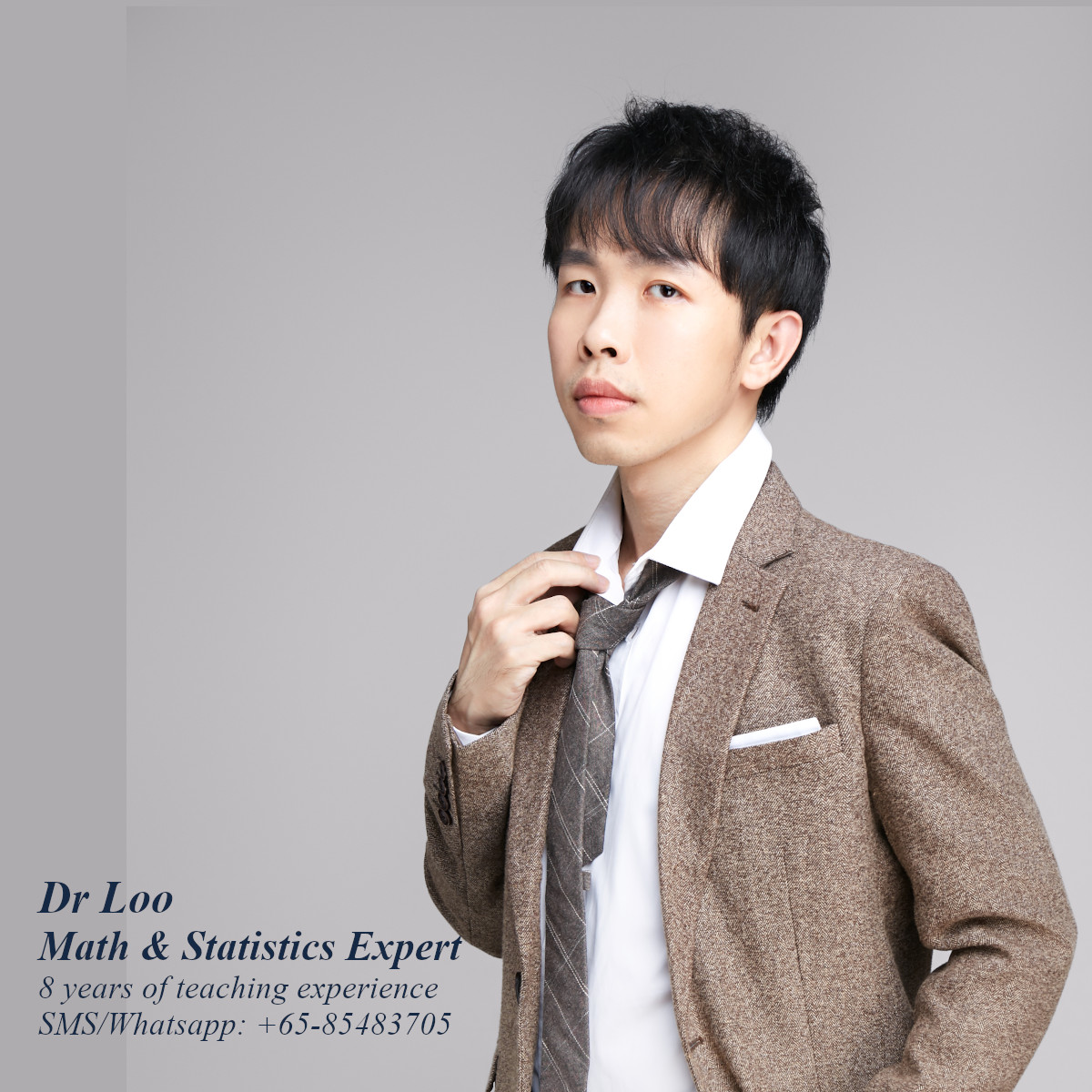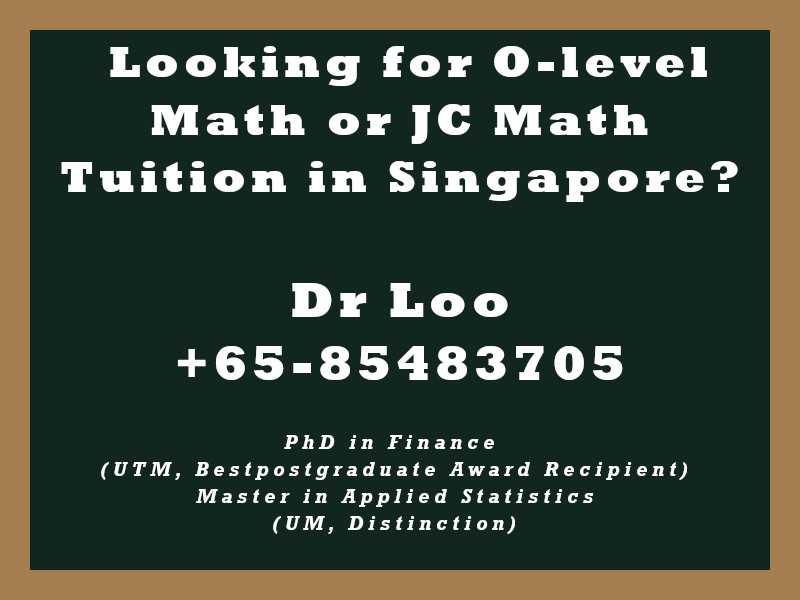+65-85483705

# Mensuration (Area)

Useful Formulae

These are some of the formulae you will be learning in the math class for calculating area.

## Other surface areas

 Shape Formula Description Cube 6 x Length x Width A cube is made up of of six square surfaces with same area. Cuboid (2 x Height x Width) + (2 x Height x Base) + (2 x Base x Width) Each pair of triangles have same area, total three pairs. Cylinder (solid) 2Πr 2 + 2Πr x height of the cyclinder Cone (solid) Πr 2 + Πrl l refers to slant height Sphere 4Πr 2

## Surface Area

 Shape Formula Square l x w Rectangle l x w Triangle 1/2 x b x h Parallelogram b x h Trapezium 1/2 x (Base Length + Top Length) x h Circle Πr 2

l = Length

w = Width

b = Base

h = Height

## About the Singapore Math Tutor - Dr Loo

I am a PhD holder with 9 years of teaching experience on teaching quantitative subjects at secondary school and university levels. My expertise are mathematics, statistics, econometrics, finance and machine learning.

Currently I do provide consultation and tuition for math tuition in Singapore. If you are looking for secondary math tutor in Singapore, JC H2 math tutor or statistics tutor in Singapore, please feel free to contact me at +65-85483705 (SMS/Whatsapp).

Among the Singapore math tuition that I provide, this includes Singapore secondary math tuition (E-math tuition & A-math tuition) and also JC Math Tuition (H1 Math Tuition & H2 Math Tuition). On the other hand, my statistics tuition in Singapore mostly focus on pre-university level until postgraduate level. For those who are looking for online tutoring service, the online O-level math tuition and online A-level math tuition are also available.## For those who are looking for math tution in Singapore

Need help with this topic? I do provide mathematics home tuition in Singapore for O-level math and also JC H2 math. In addition, online math tutoring is available as well. Feel free to contact me if you would like to know further.